# Crystal Field Theory

## Introduction

In this tutorial let’s learn about the Crystal field theory. Before moving into the definition of Crystal field theory lets have an overall understanding of certain terminologies which will help us in better understanding of this concept.

## Degenerate Energy Levels

If the same energy level is obtained by more than one quantum mechanical state, then the energy levels are said to be degenerate.

### Transition Metals

Transition metals have an empty d orbital. The d orbital split into two energy sublevels, the electron moving between these gives them their properties.

Few examples of transaction metals are Copper, Iron, Manganese, etc.

### Ligand

If an atom, a molecule, or an ion which is capable of donating a pair of electrons to central metal and forms a coordinate bond with it.

Now let’s have an overview of Crystal field theory −

“The collaboration between a transition metal and ligands develops from the attraction between the positively charged metal cation and the negative charge on the non-bonding electrons of the ligand”.

Ligands are considered as point charges in case of anions or point dipoles in case of neutral molecules. The five d orbitals in an isolated gaseous metal atom have same energy, i.e., they are degenerate. This degeneracy is kept, if a spherically balanced field of negative charges surrounds the metal atom. However, when this negative field is due to ligands in a complex, it becomes unbalanced, and the degeneracy of the d orbitals is lifted which results in splitting of the d orbitals.

The form of separation depends upon the nature of the crystal field.

Crystal field splitting into

• Octahedral Coordination Entities

• Tetrahedral Coordination Entities.

### Crystal Field Splitting into Octahedral Coordination Entities

• Six ligands are required for the octahedral complex.

• Molecular compounds with six pairs of electrons or ligands or bonds are called octahedral

• Octa means eight.

• Curious to know why molecular compounds with six pairs of electrons or bonds are called octahedral?

It's because the shape has 8 faces.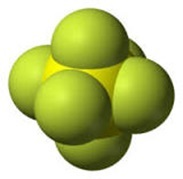Figure 1: Six Ligands

The metals which consist of d orbitals i.e., $\mathrm{dxy,\:dyz,\:dzx,\:dx^2-dy^2,\:Dz^2}$ are called 5d orbitals. These 5d orbitals present in the metals are called as degenerate energy levels. This is because all d orbitals have the same energy level.

Now, let’s see the structure of d orbitals,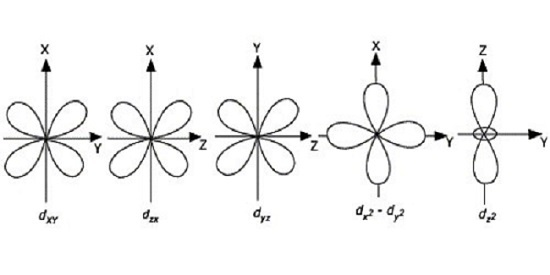Figure 2: Structure of d orbitals

As shown in the above figure,

• In dxy - the lobes are present between the x and y axis

• In dyz - the lobes are present between the y and z axis

• In dzx - the lobes are present between the z and x axis

• In $\mathrm{dx^2 – dy^2}$ - the lobes are present along the x and y axis

• In $\mathrm{dz^2}$ - the lobes are present along the z axis

The dxy, dyz, dzx are called as $\mathrm{t_{2g}}$ orbitals and $\mathrm{dx^2-dy^2,\:Dz^2}$ are called as eg orbitals. Please find the figure below for better understanding.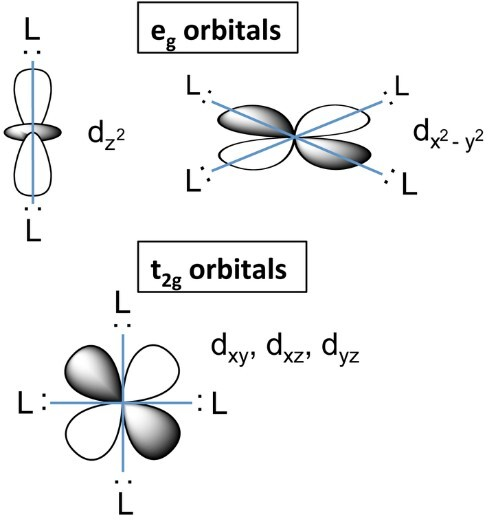*Please note all d orbitals have the same energy level

Figure 3: t2g orbitals and eg orbitals

If we see the complexes like $\mathrm{[Co(CN_6)]^{3-}\: and\:[CoF_6]^{3–}}$ fluoride ion $\mathrm{(F_6)}$ and Cyanide ion$\mathrm{(CN_6)}$ approaches the metal to form a complex.

When the ligands approach the metal to form a complex there will be a repulsion between the ligands and the metals. Whenever there is a repulsion the d orbital loses its degeneracy. When it loses its degenerate it becomes non degenerate d orbital or Average energy d orbital in spherical crystal field.

This non degenerate d orbitals split into 2 sets.

• eg set

• $\mathrm{t_{2g}}$ set.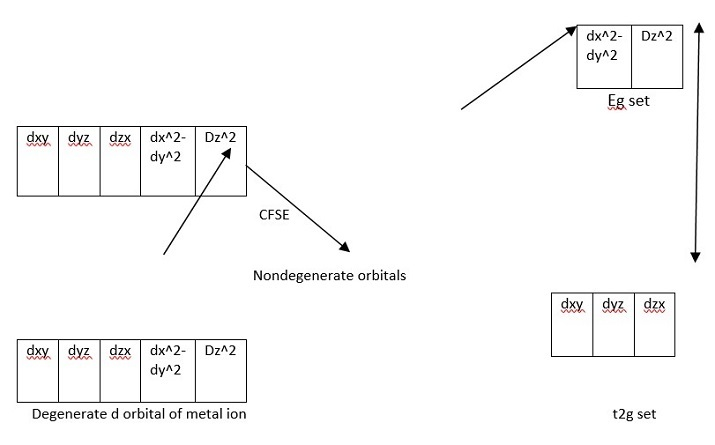Figure 4: Octahedral Complexes

In case of octahedral complexes, the ligands approached along the axis. As explained in Figure 2, eg set are along the axis. As ligands and eg lobes are along the axis the repulsion is high in eg set and the energy levels are raised. Since t2g set experience very less repulsion their energy levels are lowered. This splitting is called as the crystal field splitting energy(CFSE) or the crystal field stabilization energy.

The energy difference between the eg set and $\mathrm{t_{2g}}$ set is called as Crystal field splitting energy and is represented as $\mathrm{\Delta o}$.

Here,

∆ stands for crystal field splitting energy and

O stands for octahedral complexes.

Thus,

The energy level of eg is increased by +3/5∆o = value of eg is +0.6∆o

The energy level of $\mathrm{t_{2g}}$ is decreased by -2/5∆o = value of $\mathrm{t_{2g}}$ is -0.4∆o

### Crystal field Splitting into Tetrahedral Coordination Entities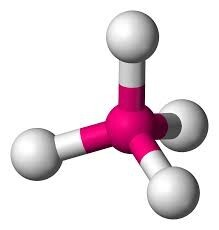Figure 5: Tetrahedral complexes

In case of tetrahedral complexes, the number of bonds formed between metal and ligand is four. So, coordination number is also four.

Coming to the Tetrahedral complexes, the ligands approach across the orbits.

As ligands and t2 lobes are across the axis the repulsion is high in t2 set and the energy levels are raised. Since e set experience very less repulsion their energy levels are lowered as shown in the figure 6 below.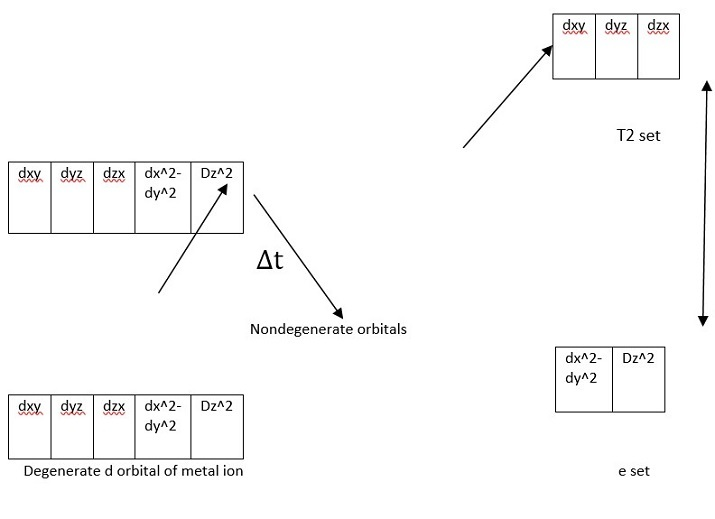Figure 6: Tetrahedral Complexes

The energy difference between t2 set and e set is represented as ∆t

Where, t stands for tetrahedral complexes.

The energy level of t2 is increased by +2/5∆o = value of eg is +0.4∆t

The energy level of e is decreased by -3/5∆t = value of t2g is -0.6∆t

(Note: Since tetrahedral complexes lack symmetry, ‘g’ subscript is not used with energy levels)

## FAQs

Q1. Who and when was the crystal field theory discovered?

Ans. Hans Bethe, a physicist, developed the crystal field theory (CFT) for crystalline solids in 1929.

Q2. What are the advantages of Crystal field theory?

Ans.

• This theory can illustrate stability of complexes. More crystal field splitting energy, more will be the stability.

• This theory can explain the color and spectra of complexes.

• This theory accounts for the magnetic properties of complexes.

Q3. What are the application of CFT?

Ans. The color for a coordination complex can be predicted using the Crystal Field Theory (CFT). Knowing the color can have a number of useful applications, such as the creation of pigments for dyes in the textile industry.

Updated on: 23-Aug-2023

233 Views